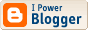## Book Review: The Computer as Crucible: An Introduction to Experimental Mathematics

Keith Devlin and Jonathan Borwein. AK Peters, 2008, ISBN-13: 978-1568813431

Whenever a book’s preface states its aims, a natural question to ask is whether it succeeds in meeting them. Keith Devlin and Jonathan Borwein, two mathematicians with expertise in different mathematical fields but with a common interest in experimental mathematics, begin this book by saying:

“Our aim in writing this book was to provide a short, readable account of experimental mathematics. It is not intended as a textbook to accompany a course…In particular, we do not aim for comprehensive coverage of the field; rather, we pick and choose topics and examples to give the reader a good sense of the current state of play in the rapidly growing field of experimental mathematics”

The sleuth-like style and lucid writing certainly make this book an enjoyable read. Many explanations are framed by relevant historical context and tales of mathematicians whose use of experimental mathematics helped them gain insights into difficult problems. Although it was never intended to be a course textbook, it could be used as a supplementary text. Many of the chapters are short, and should be viewed as aperitifs.

Chapter 1 deals with the important question “What is Experimental Mathematics?”. In the authors’ own words,

“Experimental mathematics is the use of a computer to run computations – sometimes no more than trial-and-error tests - to look for patterns, to identify particular numbers and sequences, to gather evidence in support of specific mathematical assertions that may themselves arise by computational means, including search”.

Broadly speaking, it is the use of computers in mathematics as tools in their own right, not simply as numerical calculation aids, “...experimentation is regarded as a significant part of mathematics in its own right…”.

What kind of experimentation? Here are some of the things described in this book:

• Symbolic computation using a computer algebra system such as Maple or Mathematica
• Data Visualisation
• Integer-relation algorithms like PSLQ
• High precision integer and floating point arithmetic
• High precision evaluation of integrals and summation of infinite series
• Identification of functions based on their graph characteristics

It would be very easy to fall into the belief that great mathematicians pluck profound and deep results out of thin air, but some of the mathematical greats (Gauss, Euler, Fermet, Riemann...) were confirmed experimenters who would spend many hours carrying out calculations in order to discover new mathematical avenues worth pursuing. The 72 year old Gauss recounted in a letter to the astronomer, Johann Encke, that as a young boy of 15, armed with a table of logarithms he ‘frequently spent an idle quarter of an hour to count another chiliad here and there’ , which led to his estimate of the density of prime numbers; “..Gauss was very clearly an ‘experimental mathematician’ of the first order.”

Chapter 2 gives a brief introduction to the PSLQ algorithm, an integer relation algorithm developed by Helaman Ferguson. Given any real coefficients a0, a1 ,..., an and a precision ε, an integer relation algorithm uses high-precision arithmetic to find integer coefficients λ0, λ1, λ2, ..., λn such that λ0 ≠ 0 and

0a0 + λ1a1 + ... + λnan| < ε

or else it tells you such expression exists within a ball of a given radius about the origin.

Chapter 3 (What Is That Number?) introduces Inverse Symbolic Calculators as tools to recognise numbers, and combined with Sloane’s online Encyclopedia of Integer Sequences, describes a technique for determining closed forms of sequences.

I have more than a passing interest in Riemann’s zeta function, the topic of Chapter 4 (The Most Important Function in Mathematics); I found it interesting though perhaps a little short. I particularly liked the quote about British soccer player, Wayne Rooney, contrasting him with David Beckham: “There is more chance of him [Rooney] proving Riemann’s Hypothesis than wearing a sarong”!

I’m certain physicists will find chapter 5 (Evaluate the Following Integral) interesting, especially given the authors’ collaborations in computing closed forms of definite integrals arising in physics.

Chapter 9 (Take It to the Limit) contains 3 worked examples of finding closed forms for infinite sums, and Chapter 10 (Danger! Always Exercise Caution When Using the Computer) contains sobering stories and examples of some of the pitfalls faced by experimental mathematicians. Here is one:

A computer algebra system (CAS) will discover that I1 = I2 = I3 = ... = I7 = π/2 but I8 = 0.499999999992646π

On finding this, the authors suspected a bug in the CAS software. But there is no bug!

The book provides tantalising examples and suggestions to whet the reader’s appetite in the form of an ‘Explorations’ section at the end of each chapter, not exactly exercises but there is a corresponding ‘Answers and Reflections’ chapter at the end of the book. Interested readers will find many of these topics expanded upon in .

I thoroughly enjoyed reading this short introduction to experimental mathematics. It will no doubt appeal to a broad mathematical audience, both professional and amateur alike. If I have one complaint, well more of a request, it would be a much longer chapter on evaluating definite integrals! But then, in the words of G. H. Hardy, “I could never resist a definite integral” .

### References

 David Bailey et al. (2007). Experimental Mathematics in Action. AK Peters, MA.

 Macdonald, H. S. (1999). The Beauty of Geometry: Twelve Essays. Dover Publications.

 Havil, J. (2003). Gamma: Exploring Euler’s Constant. Princeton UP.

[This review appeared in the July 2009 issue of the Australian Mathematical Society’s Gazette.]

<< Home###### Contact

MSN, Email: mitch døt wheat at gmail.com